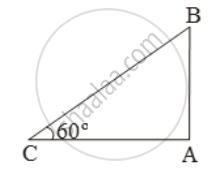# The altitude of the sun at any instant is 60º. Find the height of the vertical pole that will cast a shadow of 30 m. - Mathematics

Sum

The altitude of the sun at any instant is 60º. Find the height of the vertical pole that will cast a shadow of 30 m.

#### Solution

Let AB be the pole and AC be its shadow.

Then, θ = 60º and AC = 30 m.\therefore \frac{AB}{AC}=\text{tan }60^\text{o}

\Rightarrow \frac{AB}{30}=\sqrt{3}\Rightarrow AB=30\sqrt{3}m

Concept: Heights and Distances
Is there an error in this question or solution?